# Electronics and Communication Engineering - Power Electronics

### Exercise :: Power Electronics - Section 5

46.

The turn on characteristics of a power transistor can be assumed to be linear, as shown in the below figure . During turn on time T, voltage reduces linearly from V to 0 and current rises linearly from 0 to I. The energy loss during time T is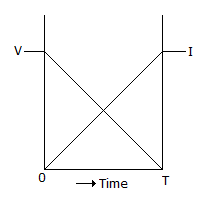A. VIT B.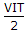C.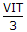D.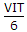Explanation:

No answer description available for this question. Let us discuss.

47.

The circuit in figure is for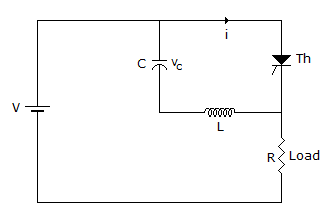A. resonant commutation B. self commutation C. load commutation D. auxiliary commutation

Explanation:

No answer description available for this question. Let us discuss.

48.

A single phase half bridge inverter required to feed R-L loads needs

 A. 2 thyristors B. 4 thyristors C. 2 thyristors and two diodes D. 4 thyristors and 4 diodes

Explanation:

No answer description available for this question. Let us discuss.

49.

Typical values of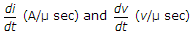for an SCR are in the range of

 A. 1 to 10 B. 25 to 500 C. 0.1 to 1 D. 1000 to 2000

Explanation:

No answer description available for this question. Let us discuss.

50.

When a dc chopper feeds an RLE load, the load current, during steady state operation

 A. remains constant B. varies between maximum and minimum values C. may remain constant or vary D. is constant if R is constant